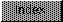```

NAME
IEEESPDiv -- divide one single precision IEEE by another

SYNOPSIS
x   = IEEESPDiv(  y  ,  z  );
d0                d0    d1

float   x,y,z;

FUNCTION
Compute x = y / z in IEEE single precision.
Note that the Motorola fast floating point Div routine reverses
the order of the arguments for the C interface, although the
dividend is still in d0 and the divisor is in d1.

INPUTS
y -- IEEE single precision floating point value
z -- IEEE single precision floating point value

RESULT
x -- IEEE single precision floating point value

BUGS